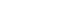# Lesson Tutor: Printable Wordsearch for Music Time and Tone.

Music Notation: Time and Tone Vocabulary and Definitions
Printable Wordsearch 1

 a c c e l e r a n d o e m a t l o v p o c o n i n e t s e a m b f l a r g o m d r r m a m c l e g a t o i g o r p e o i a r d a n m i o j i o n n o h a o o i z r d a f o r t e u t r n o g o n m t n s i l a t u w e h i e e w a o m s e e a l o s m c k m i e e m n l l j s o t s e r p c u d t a m i n o r e y e a s o z z e m e s t o r a v i z m e n o t a b u r c i c t i m e s p r e s s i v o

Instructions
1. Print page 1 to 1 only
2. Find and circle the terms described below.
 1. smooth and connected: ___________________ 2. sweetly: ___________________ 3. a little: ____________________ 4. very fast: __________________ 5. expressively: ______________________ 6. return to previous time (2 words): _______________ 7. pp :_________________ 8. extrememly slow and solemn: ______________ 9.  f  : ____________ 10 very: _______________ 11 fast and cheerful: _______________ 12 also known as 4/4 time (2 words): _____________ 13 ____ forte: moderately loud: __________________ 14: ____________________